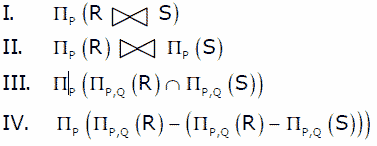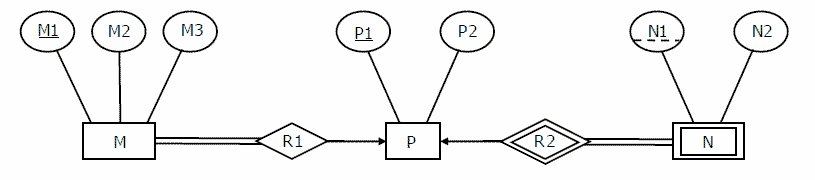# Database Management Systems | Set 7

• Difficulty Level : Medium
• Last Updated : 27 Mar, 2017

Following questions have been asked in GATE 2008 CS exam.

1) Let R and S be two relations with the following schema
R (P,Q,R1,R2,R3)
S (P,Q,S1,S2)
Where {P, Q} is the key for both schemas. Which of the following queries are equivalent?(A) Only I and II
(B) Only I and III
(C) Only I, II and III
(D) Only I, III and IV

In I, Ps from natural join of R and S are selected.
In III, all Ps from intersection of (P, Q) pairs present in R and S.
IV is also equivalent to III because (R – (R – S)) = R ∩ S.
II is not equivalent as it may also include Ps where Qs are not same in R and S.

2) Consider the following ER diagram.The minimum number of tables needed to represent M, N, P, R1, R2 is
(A) 2
(B) 3
(C) 4
(D) 5

See http://geeksquiz.com/gate-gate-cs-2008-question-82/ for explanation.

3) Which of the following is a correct attribute set for one of the tables for the correct answer to the above question?
(A) {M1, M2, M3, P1}
(B) {M1, P1, N1, N2}
(C) {M1, P1, N1}
(D) {M1, P1}

4) Consider the following relational schemes for a library database:
Book (Title, Author, Catalog_no, Publisher, Year, Price)
Collection (Title, Author, Catalog_no)

with in the following functional dependencies:

```I. Title Author --> Catalog_no
II. Catalog_no --> Title Author Publisher Year
III. Publisher Title Year --> Price ```

Assume {Author, Title} is the key for both schemes. Which of the following statements is true?
(A) Both Book and Collection are in BCNF
(B) Both Book and Collection are in 3NF only
(C) Book is in 2NF and Collection is in 3NF
(D) Both Book and Collection are in 2NF only